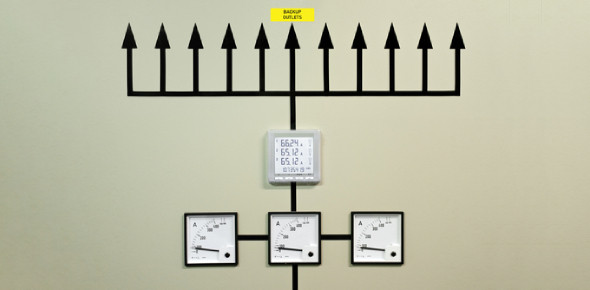# Capacitive Reactance MCQ Questions With Answers

18 Questions | Attempts: 420
ShareSettingsDo you know how to find the resistance and capacitance of a capacitor? How good are you at solving capacitive reactance MCQ questions? Play this quiz and answer the questions based on capacitive reactance and check your skills. The capacitive reactance is defined as the total opposition to the alternating current due to a capacitor and is denoted by Xc. Here, you have to solve numerical based on Xc. Take the quiz and see how easily you can solve them.

• 1.
As the size of the plates in a capacitor increases, all other things being equal,
• A.

The value of XC increases negatively.

• B.

The value of XC decreases negatively.

• C.

The value of XC does not change.

• D.

We cannot say what happens to XC without more data.

• 2.
If the dielectric material between the plates of a capacitor is changed, all other things beingequal,
• A.

The value of XC increases negatively.

• B.

The value of XC decreases negatively.

• C.

The value of XC does not change.

• D.

We cannot say what happens to XC without more data.

• 3.
As the frequency of a wave gets lower, all other things being equal, the value of XC for acapacitor
• A.

Increases negatively

• B.

Decreases negatively

• C.

Does not change

• D.

Depends on the current

• 4.
What is the reactance of a 330-pF capacitor at 800 kHz?
• A.

−1.66 Ω

• B.

−0.00166 Ω

• C.

−603 Ω

• D.

−603 kΩ

• 5.
Suppose a capacitor has a reactance of −4.50 Ω at 377 Hz. What is its capacitance?
• A.

9.39 µF

• B.

93.9 µF

• C.

7.42 µF

• D.

74.2 µF

• 6.
Suppose a 47-µF capacitor has a reactance of −47 Ω. What is the frequency?
• A.

72 Hz

• B.

7.2 MHz

• C.

0.000072 Hz

• D.

7.2 Hz

• 7.
Suppose a capacitor has XC =−8800 Ω at f = 830 kHz. What is C?
• A.

2.18 µF

• B.

21.8 pF

• C.

0.00218 µF

• D.

2.18 pF

• 8.
Suppose a capacitor has C = 166 pF at f = 400 kHz. What is XC ?
• A.

−2.4 kΩ

• B.

−2.4 Ω

• C.

−2.4 × 10−6 Ω

• D.

−2.4 MΩ

• 9.
Suppose a capacitor has C = 4700 µF and XC =−33 Ω. What is f?
• A.

1.0 Hz

• B.

10 Hz

• C.

1.0 kHz

• D.

10 kHz

• 10.
Each point in the RC plane
• A.

Corresponds to a unique inductance

• B.

Corresponds to a unique capacitance

• C.

Corresponds to a unique combination of resistance and capacitance

• D.

Corresponds to a unique combination of resistance and reactance

• 11.
If R increases in an RC circuit, but XC is always zero, the vector in the RC plane will
• A.

Rotate clockwise

• B.

Rotate counter clockwise

• C.

Always point straight toward the right

• D.

Always point straight down

• 12.
If the resistance R increases in an RC circuit, but the capacitance and the frequency arenonzero and constant, then the vector in the RC plane will
• A.

Get longer and rotate clockwise

• B.

Get longer and rotate counter clockwise

• C.

Get shorter and rotate clockwise

• D.

Get shorter and rotate counter clockwise

• 13.
Each complex impedance value R − jXC
• A.

Represents a unique combination of resistance and capacitance

• B.

Represents a unique combination of resistance and reactance

• C.

Represents a unique combination of resistance and frequency

• D.

All of the above are true.

• 14.
In an RC circuit, as the ratio XC /R approaches zero, the phase angle
• A.

Approaches −90°.

• B.

Approaches 0°

• C.

Stays the same

• D.

Cannot be found

• 15.
In a purely resistive circuit, the phase angle is
• A.

Increasing

• B.

Decreasing

• C.

• D.

−90

• 16.
If XC /R =−1, then what is the phase angle?
• A.

• B.

−45°

• C.

−90°

• D.

Impossible to find because there’s not enough data given

• 17.
Suppose an RC circuit consists of a 150-pF capacitor and a 330-Ω resistor in series. What isthe phase angle at a frequency of 1.34 MHz?
• A.

−67.4°

• B.

−22.6°

• C.

−24.4°

• D.

−65.6°

• 18.
Suppose an RC circuit has a capacitance of 0.015 µF. The resistance is 52 Ω. What is thephase angle at 90 kHz?
• A.

−24°

• B.

−0.017°

• C.

−66°

• D.

None of the above

## Related TopicsBack to top
×

Wait!
Here's an interesting quiz for you.